# Basics of Neural Network - Perceptron Model

dougieduk·2022년 4월 10일
0

# Biological Neurons

• Dendrites : inputs going into nucleus
• Nucleus : does the calculation
• Axon : passes output to other neurons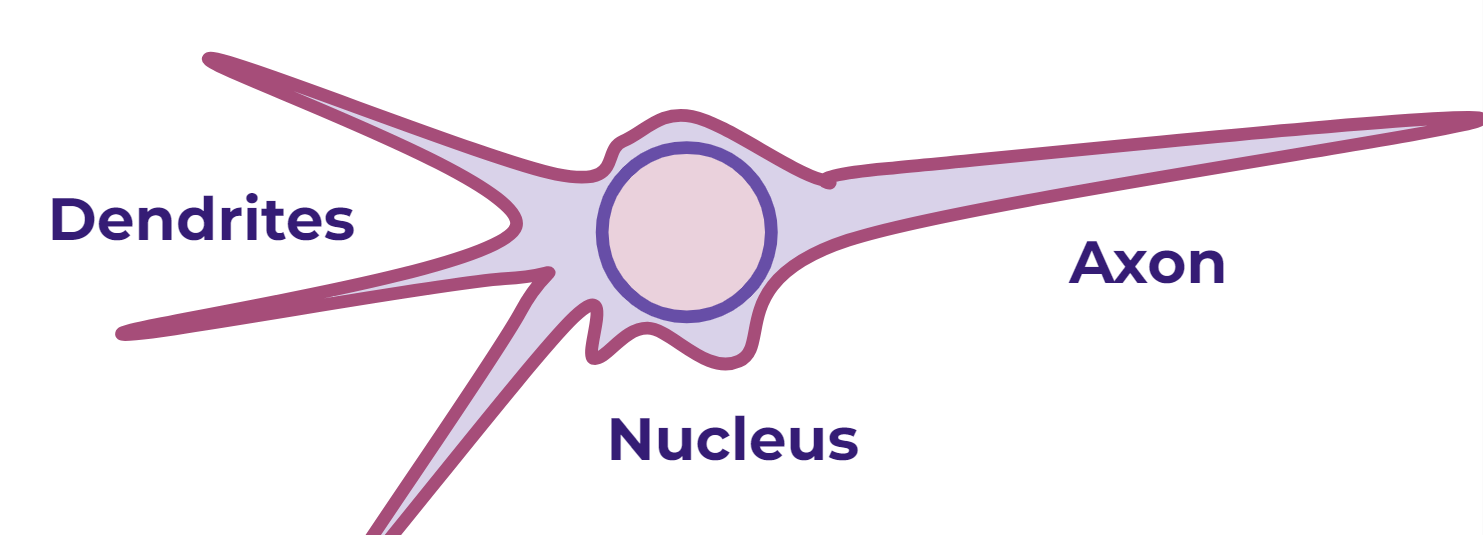# Perceptron Models

• Neural Networks mimic this model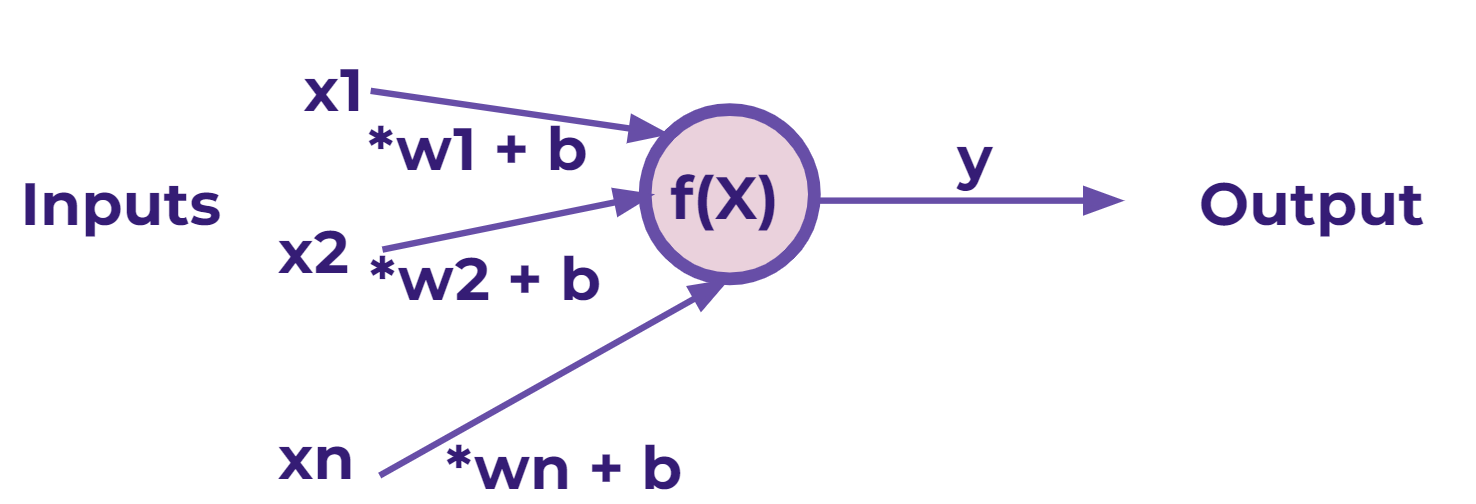• Generalized formula for perceptrons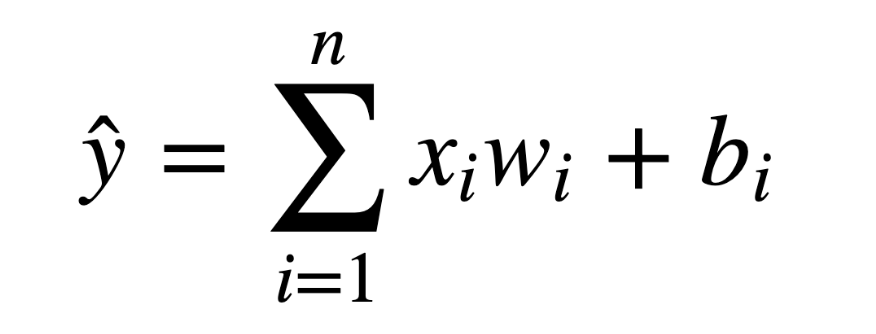# Neural Networks

• A single perceptron won’t be enough to learn complicated systems.
• To build a network of perceptrons, we can connect layers of perceptrons, using a multi-layer perceptron model.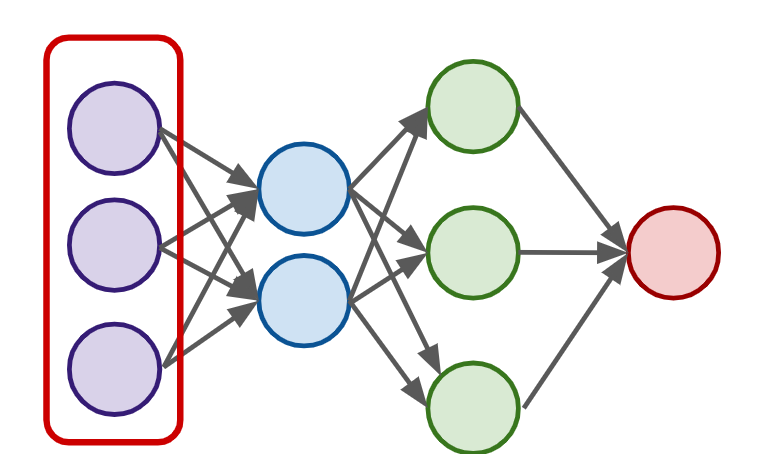• Input Layer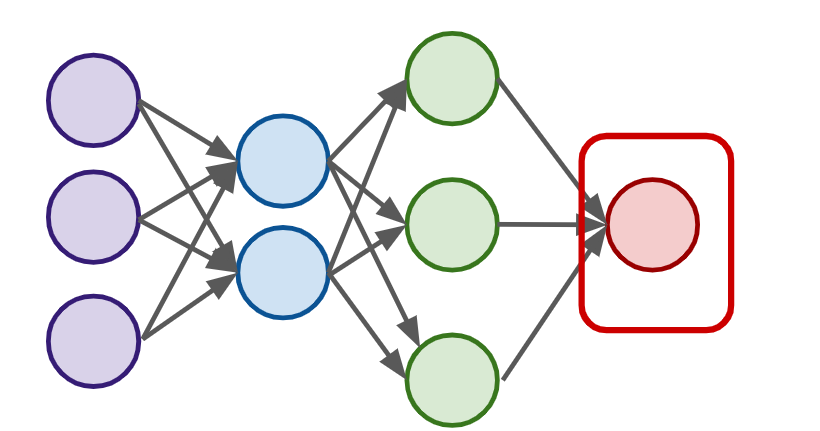• Output Layer : final estimate of the output
- There could be multiple output layers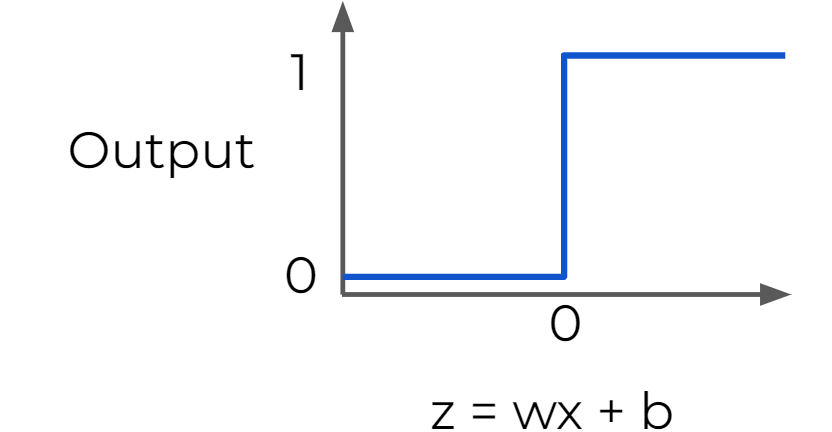• Hidden Layer : the layers in the middle
- Deep Network : 2 or more hidden layers
- Might be difficult to interpret

# Activation Functions

• z = x*w + b : basic formula for each perceptron
- w : how much weight or strength to give the incoming input
- b : an offset value, making x*w have to reach a certain threshold before having an effect

• Activation Function (f(z) or X ) : sets boundaries to output values from the neuron

• Step Function : Useful for classification
- a strong function - small changes aren't reflected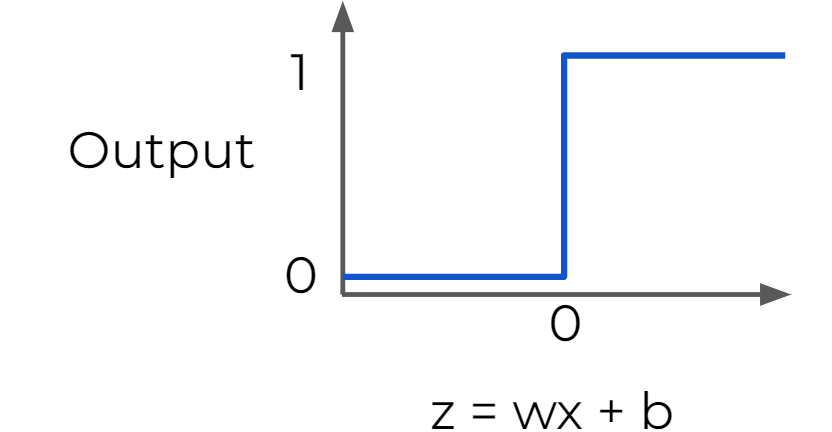• Sigmoid Function : moderate form of a step function
- more sensitive to small changes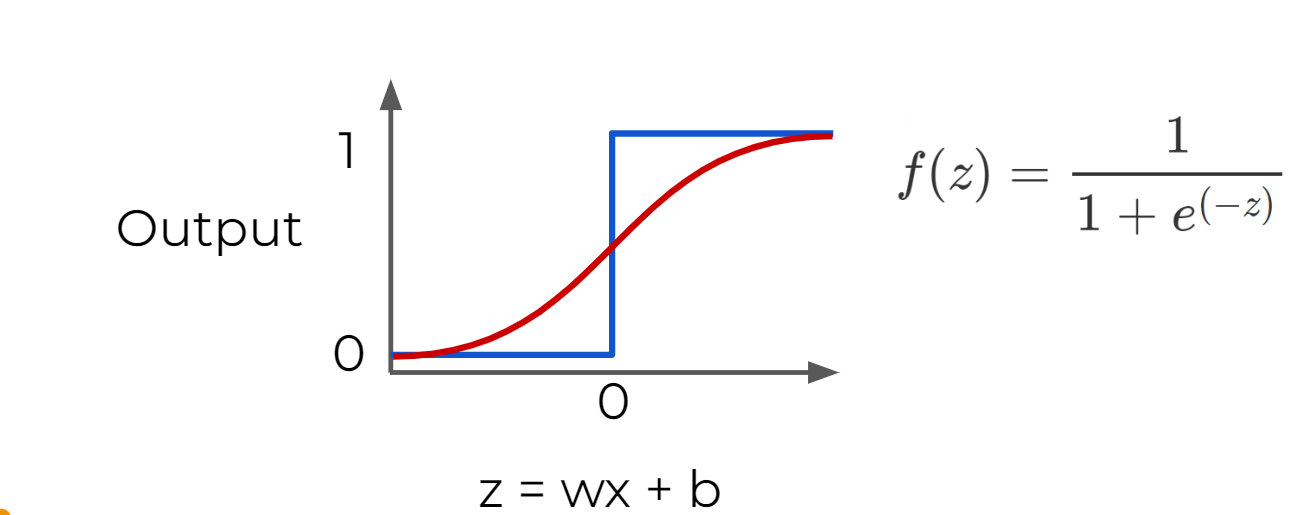• Hyperbolic Tangent : outputs between -1 and 1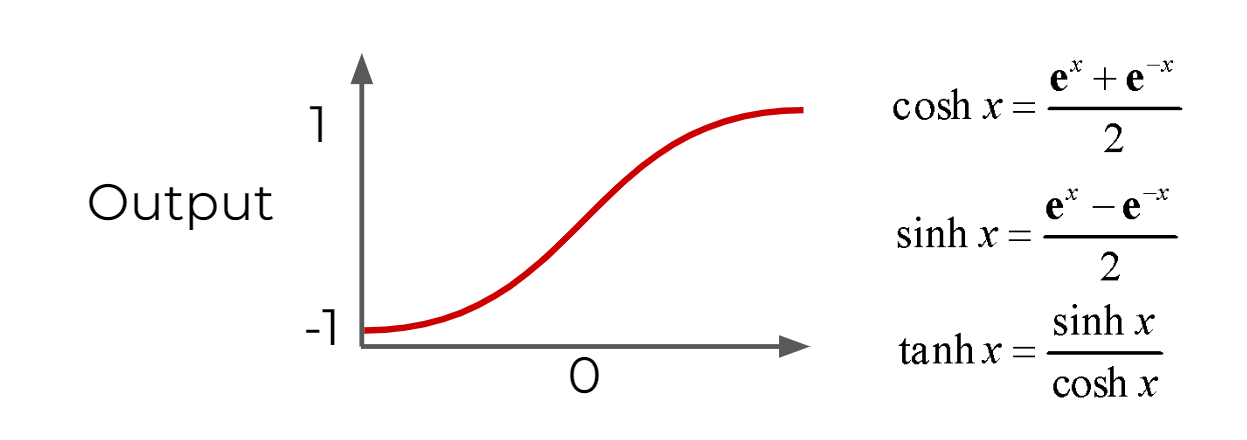• Rectified Linear Unit (ReLU) : max(0,z)
- deals with the issue of vanishing gradient
- usually used as the default activation function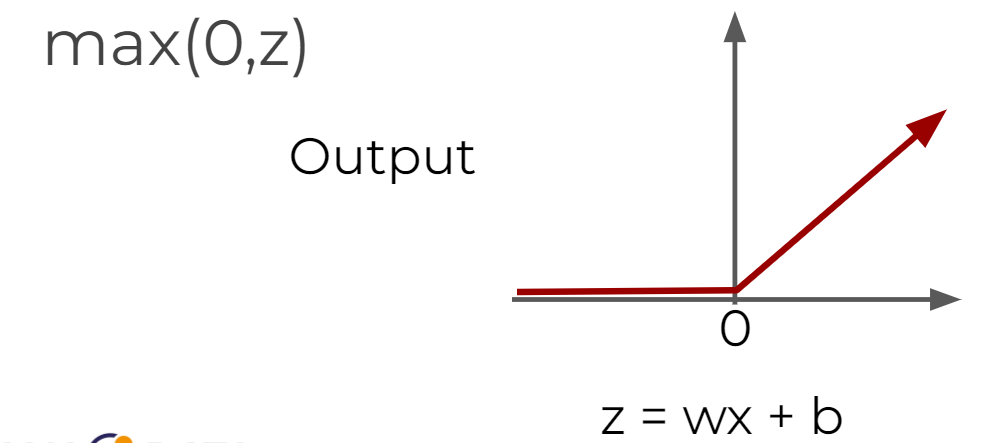# Multiclass Classifications

• There are 2 types of multi-class situations:
- Non-exclusive classes : a data point can have multiple classes/ categories assigned to it (e.g. tagging photos )
- Mutually Exclusive Classes : only one class per data point
• For multiclass classifications, arrange multiple output layers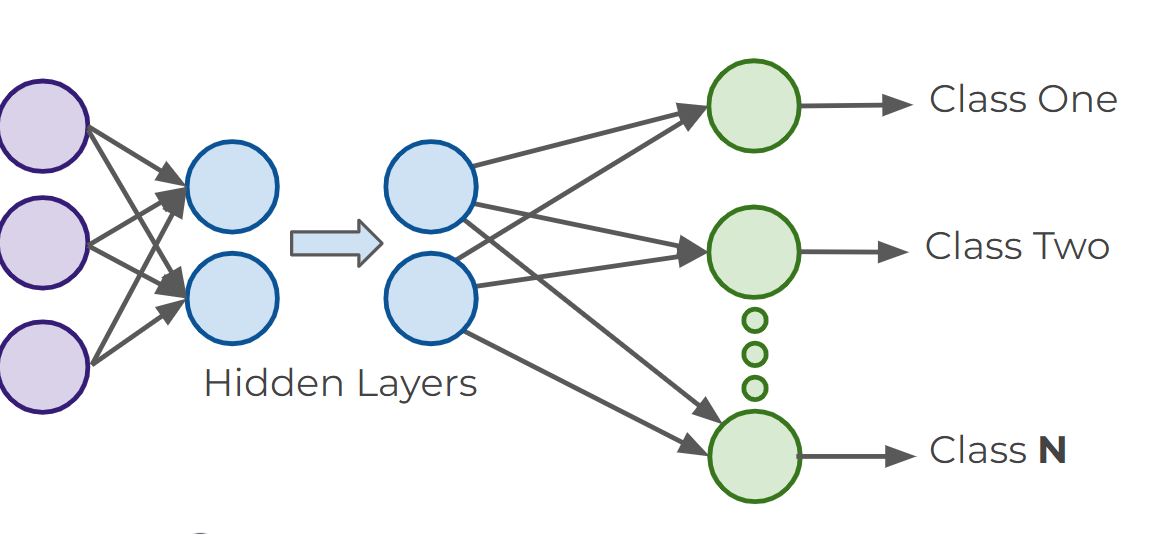• One-hot encoding : how to turn classes into vectors
- Mutually Exclusive Classes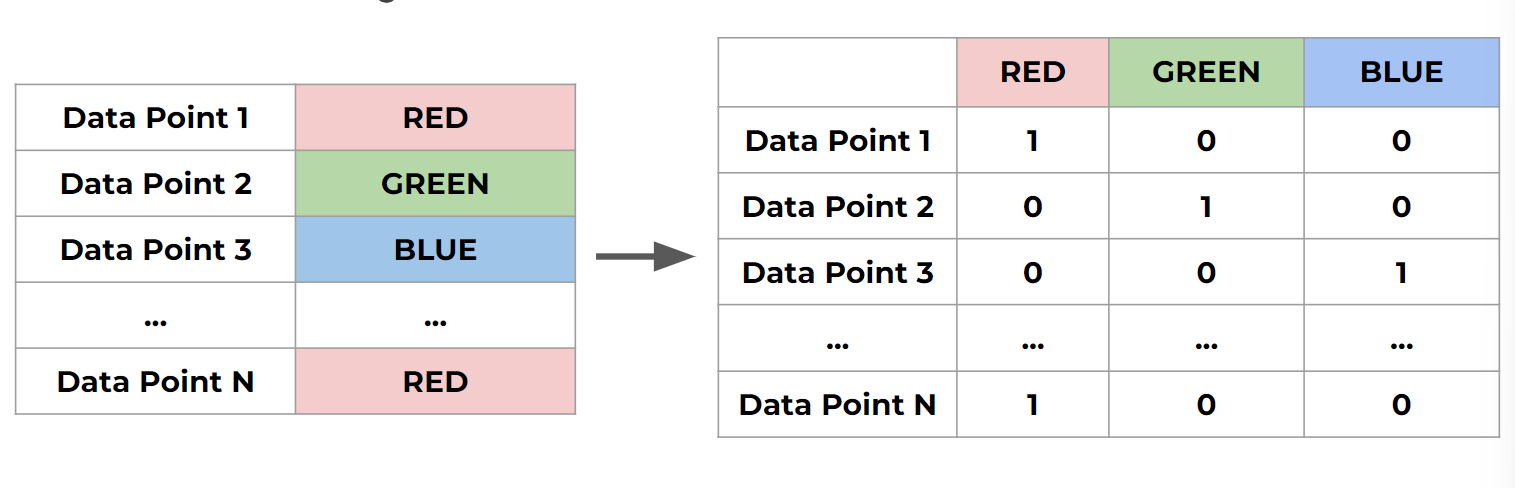- Non-exclusive classes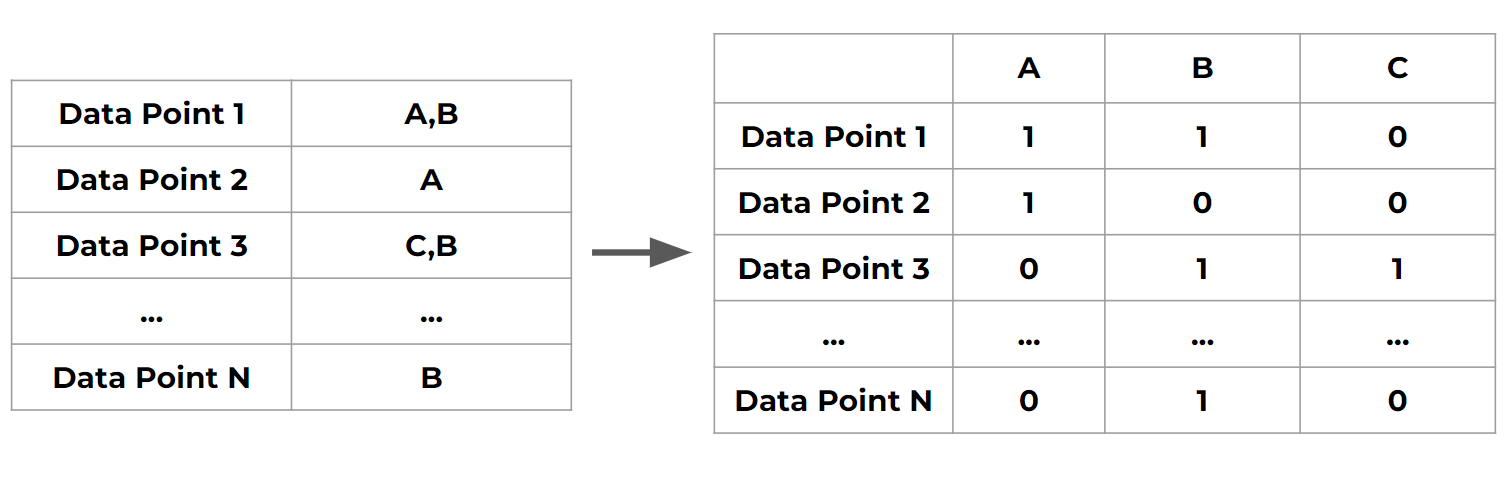• Activation functions for multiclass classification (Non-exclusive)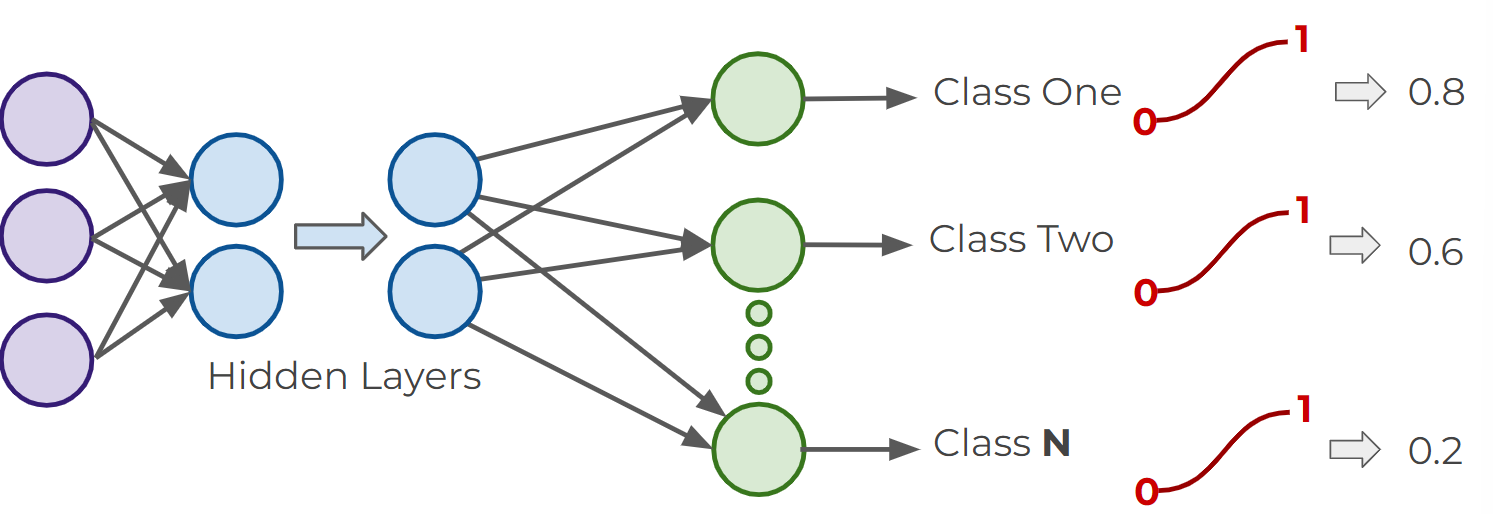• Activation functions for multiclass classification (Exclusive)
- Softmax Function : the target class chosen will have the highest probability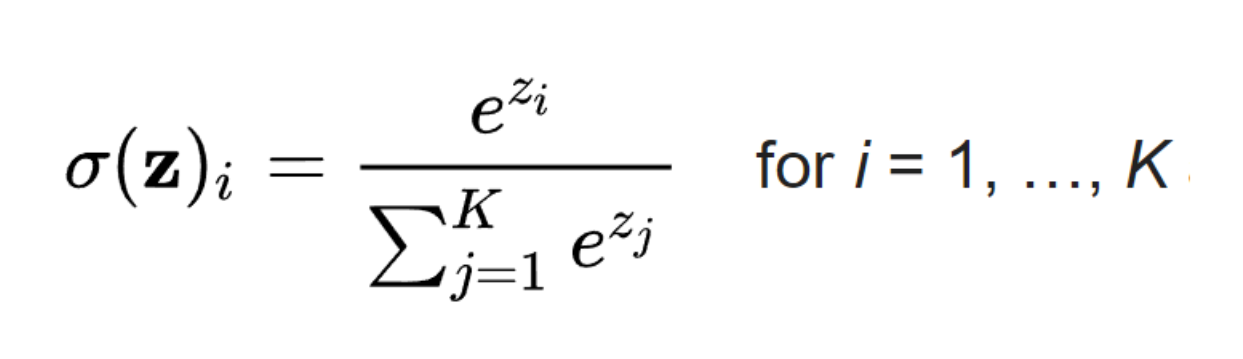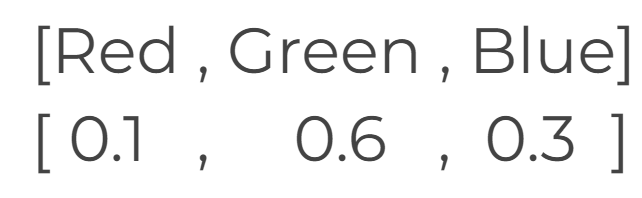# Cost Functions and Gradient Descent

• Notations
- ŷ  : estimation of what the model predicts the label to be
- y : true value
- a : neuron's prediction

• Cost Function
- must be an average so it can output a single value
- Used to keep track of our loss/cost during training to monitor network performance

- aL is the prediction at L layer
- Why do we square it?

• punish large errors
• keeps everything positive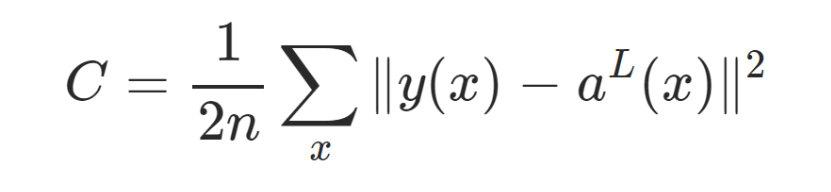• Generalization of cost function
- W is our neural network's weights, B is our neural network's biases, Sr is the input of a single training sample, and Er is the desired output of that training sample.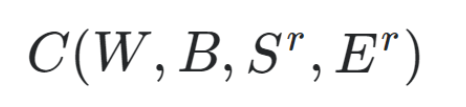• Gradient Descent : find the w values that minimizes the cost
- Learning Rate : how much you should move each time

• Larger learning rates result in overshooting but lower computing rate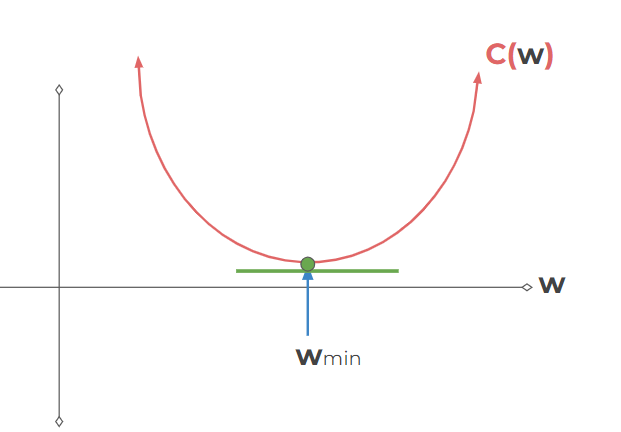• Gradient : derivative for N-dimensional Vectors
∇C(w1,w2,...wn)

• Cross Entropy Loss Function : for classification problems
- binary classfication :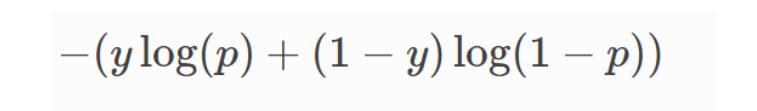- multi class classification :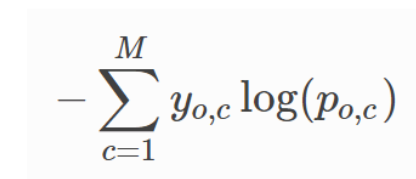# Back Propagation

• Usage of derivatives : find out how sensitive is the cost function to changes in w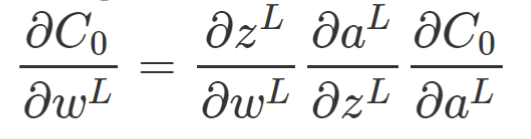- repeat the same for bias
• Back Propagation Process :
- Step 1 : use input x to set the activation function a for the input layer & repeat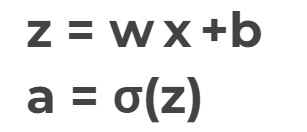- Step 2 : For each layer, compute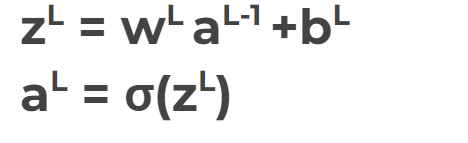- Step 3 : compute error vector